# CLASS 12 CHEMISTRY CHAPTER 6-GENERAL PRINCIPLES AND PROCESSES OF ISOLATION OF ELEMENTS

Question1. Explain why hydro-metallurgy can extract copper , but cannot extract zinc.

Solution :
Copper has a higher reduction potential than zinc or iron. Thus, in hydro-metallurgy iron and zinc can be used to displace copper from its solution.
Fe(s) + Cu2+(aq)

⇒ Fe2+ (aq) + Cu(s)
However, in-order to displace zinc a metal more reactive than it is required e.g. K, Ca , etc. The problem arising now is that all these metals react with water to liberate hydrogen gas. Thus, they cannot be used for the extraction of zinc using hydro-metallurgy.

Question2. What role does a depressant play in the process of froth floatation ?

Solution :
The role of a depressant in forth floatation is to set apart two sulphide ores through selective prevention of one ore from frothing. E.g. NaCN is used to separate two sulphide ores , PbS and ZnS. NaCN forms a complex, Na2 [Zn( CN )4], with zinc on the surface of zinc sulphide, thus selectively preventing ZnS from frothing.
4NaCN + ZnS ⇒ Na2 [Zn( CN )4] + Na2S

Question3. Why is it more difficult to extract copper from pyrites than it is to extract copper from its oxide ore ?

Solution :
Hydrogen sulfite and carbon disulfite (CS2) has a larger ∆fG (Gibbs free energy of formation) than copper (I) sulfite. Thus, C and H2S will not be able to reduce Cu2S to Cu.
Whereas, ∆fG of Cu2O is larger than carbon monoxide’s , thus C can reduce copper (I) oxide to copper.
Therefore, it is more difficult to extract copper from pyrites than it is to extract from its oxide.

Question4. Write notes on :
(a) Zone refining
(b) Column chromatography.

Solution :
(a) Zone refining:
This method makes use of a principle according to which impurities are more soluble in molten metal than in a solid metal. In zone refining, a moving circular heater slowly moves over an impure metal rod/ bar. As the circular heater moves, the impurities move into the adjacent molten zone and the pure metal crystallizes out. This is repeated over several times, causing the segregation of impurities at one end of the bar. This impure end is then cut off. Boron, silicon, gallium etc. are purified this way.

(b) Column chromatography:
This is a very useful method of purifying elements present in minute amounts. Column chromatography is mostly used in order to take away those impurities which are not very different in chemical properties from the element which needs to be purified. This concept follows the principle various components of a mixture are adsorbed to different extents on an adsorbent.

Chromatography includes two phases : mobile phase and stationary phase. This phase ( stationary ) is immiscible and immobile. A column of adsorbent e.g. Al2 O3 is used as the stationary phase. The mixture to be purified is dissolved in the mobile phase which can be a liquid, gas or supercritical fluid. This is then poured onto the top of the column. The component which is more strongly adsorbed by the adsorbent takes a greater amount of time to move through it than the component that is weakly adsorbed. This way the different components of the mixture are separated as they move through the stationary phase.

Question5. At 673 K which is a more effective reducing agent, CO or C ?

Solution :
At 673 K ,CO is a more effective reducing agent because, at 673 K the value of ∆G( C,CO ) is greater than the value of ∆G( CO,CO2 ), which means that CO is more easily reduced to CO2 than C to CO.

Question6. In the process of electrolytic refining of copper what are the common elements in the anode mud ? What is the reason for their presence ?

Solution :
Selenium, silver, tellurium, platinum, antimony and gold are the common elements present in the anode mud during the electrolytic refining of copper.
As these metals are almost unreactive they are not affected during the purification method. Thus, they are present in the anode mud.

Question7. State the various reactions that take place inside the different zones of a blast furnace during iron extraction.

Solution :
Inside a blast furnace iron oxide is reduced at different temperature ranges, as given below:

At 500 – 800 K
3Fe2O3 +CO → 2Fe3O4 + CO2
Fe2O3 +CO → 2FeO + CO2

At 850 K
Fe3O4 +CO → 3FeO + CO2

At 900 – 1500 K
FeO +CO → Fe + CO2
CO2 + C → 2CO

Above 1570 K
C+ O2 → CO2
FeO +C → Fe + CO
CaCO3(s) → CaO(s) + CO2(g)
SiO2 + CaO → CaSiO3 ( slag )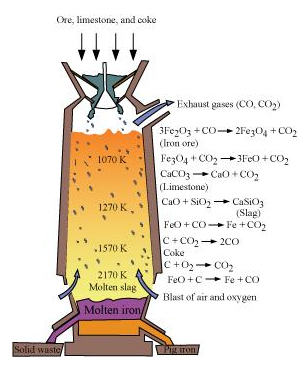Question8.Write the chemical reactions involved in zinc extraction from zinc blend.

Solution :
The various steps involved in zinc extraction are :

(1) Concentration of ore:
Firstly, to remove the impurities of the ore, zinc blende is crushed and concentration is done by froth floatation.

(2) Conversion to oxide (Roasting):
In this step ZnS is roasted in excess of air in a furnace at a temperature below the melting point of zinc. This results in the formation of zinc oxide.

2ZnS + 3O2 ⇒ 2ZnO + 2SO2

(3) Extraction of Zn from ZnO (Reduction):
To extract zinc from zinc oxide it is mixed with powdered coke and then heated.

ZnO + C ⇒ Zn + CO

(4) Electrolytic Refining:
In this method, impure zinc becomes the anode while the cathode is a pure copper strip. The electrolyte is an acidic solution of zinc sulphate (ZnSO4) with dilute sulfuric acid. On passing a current, pure zinc deposits on the copper strip.

Question9. What role does silica play in copper metallurgy ?

Solution :
Copper pyrites are converted to a mixture of Cu2O and FeO during roasting. Acidic silica is added during smelting to separate the FeO from the mixture. SiO2 and FeO forms FeSiO3 ( slag )which floats on the molten matter.
FeO + SiO2 ⇒ FeSiO3 (slag)

Question10. “Chromatography”, What do you understand by this term?

Solution :
Chromatography is a collective term used for a range of laboratory techniques for the purification, separation, characterization and identification of the species of a mixtures wether colored or colorless. The word has been obtained from two Greek words; ‘graphy’ meaning ‘to write’ and ‘chroma’ meaning ‘colour’. There are numerous chromatographic techniques like column chromatography, paper chromatography, gas chromatography, etc.

Question11. What is the criterion followed while selecting the stationary phase of chromatography ?

Solution :
The choice of stationary phase is done in a way that the elements of the mixture have different solubility in the phase. Thereby, different elements have different movement speed through the phase , because of which they can be separated from each other.

Question12. Write about a technique used to refine nickel ?

Solution :
Mond’s process is technique used to refine nickel. In this method, heat is supplied to nickel in the presence of carbon monoxide to produce nickel tetracarbonyl, which is a volatile complex.

Ni + 4CO ⇒ Ni( CO)4 [330k – 350k]

The nickel tetracarbonyl so obtained is then decomposed by heating it at a higher temperature (450 − 470 K) to form pure nickel.

Ni( CO)⇒ Ni + CO [450k – 470k]

Question13. How is alumina separated from silica in a bauxite ore associated with silica? Provide equations, if present.

Solution :
Separation of alumina from silica in a bauxite ore associated with silica is as follows :
Firstly, concentrated NaOH solution is used to digest the powdered ore at 473 − 523 K and at a pressure of 35 − 36 bar. This causes alumina ( Al2O3 ) and silica ( SiO) to leach as sodium aluminate and sodium silicate respectively, abandoning the impurities behind.

Al2O(s) + 2NaOH(aq) + 3H2O (f) ⇒ 2Na [ Al ( OH)4 ](aq)
Alumina sodium aluminate

SiO2 + 2NaOH(aq) ⇒ Na2SiO(aq) + H2O(l)
Silica Sodium silicate

Now, to neutralize the aluminate present in the solution, carbon dioxide gas is passed through the solution we obtained. This causes the sodium meta – aluminate to precipitate as hydrated alumina.

2Na [ Al ( OH)4 ](aq) + CO2

⇒ Al2O3.xH2O(s) + 2NaHCO3(aq)
hydrated alumina
Sodium silicate cannot be precipitated so it is filtered off. The hydrated alumina is dried, heated and filtered to obtain pure alumina.

Al2O3.xH2O(s) ⇒ Al2O3(s) + xH2O( g) [1470 k]

Question14. Differentiate between ‘calcination’ and ‘roasting’ with the help of examples.

Solution :
Calcination is the conversion of carbonate and hydroxide ores to oxides by heating them at a temperature below their melting points and in the absence or in a very limited supply of air.
For example, carbonates of Ca, Mg, Zn are turned into their respective oxides through this process.
ZnCOZnO + CO2

Fe2O3.3H2O ⇒ Fe2O3 + 3H[Heat]

Roasting is the conversion of sulfide ores into their metallic oxides by heating at a temperature below their melting points in excess presence of air.
For e.g, sulfide ores of Pb, and Zn are turned into their respective oxides through this process.
2Zns + 3O⇒ 2ZnO + 2SO2

2Pbs + 3O⇒ 2PbO + 2SO2

Question15. What is the difference between ‘cast iron’ and ‘pig iron’ ?

Solution :
Pig iron is the iron obtained from blast furnace. It is 4% carbon and contains many other impurities like Si, P, S, Mn in lesser amounts.
Cast iron is made by melting pig iron and coke with the help of a hot air blast. It has lower levels of carbon in it (3%). Unlike pig iron, cast iron is brittle and extremely hard.

Question16. What is the difference between “ores” and “minerals” ?

Solution :
Minerals are naturally available substances having metals or their compounds.
Ores are rocks and minerals from which metals are economically and conveniently obtained.
For e.g., there are a lot of minerals that contain, but zinc cannot be conveniently and economically extracted from all of them. Zinc is extracted from calamine (ZnCO3), zinc blende (ZnS), etc. Hence, these minerals qualify as ores of zinc.

Question17. Why is copper matte put in converters lined with silica ?

Solution :
Copper matte has Cu2S and some FeS. So, when a hot blast of air is blown through a molten matte placed in a silica lined converter , FeS of the matte oxidizes to FeO. This FeO combines with SiO2 ( silica ) to produce FeSiO3 (slag).

2FeS + 3O2 ⇒ 2FeO + 2SO2
FeO + SiO2 ⇒ FeSiO3

When all of the iron has been removed as slag Cu2S is oxidized to Cu2O which then undergoes a reaction with Cu2S to give copper metal.

2Cu2S + 3O2 ⇒ 2CuO2 + 2SO2
2Cu2O + Cu2S ⇒ 6Cu + SO2

Question18. In the metallurgy of aluminium, what role does Cryolite play ?

Solution :
Na3AlF6 ( Cryolite ) has two basic roles:

1. The melting point of the solution is decreased from 2323 to 1140 K due to the presence of cryolite.
2. Cryolite makes alumina a good conductor of electricity.

Question19. How is leaching done in when dealing with low grade copper ores?

Solution :
When dealing with low grade copper ores, bacteria or acids are used in the presence of air to leach the copper. In this method, copper enters the solution as Cu2+ ions :

Cu + 2H+ + ½ O2 ⇒ Cu2+ + 2H2O

The solution so obtained is then treated with H2 or scrap iron to get copper metal.

Cu + 2H+ ⇒ 2H2+ + Cu

Question20. Why isn’t zinc oxide reduction (using carbon monoxide) used to extract zinc ?

Solution :
As the standard Gibbs free energy of formation of CO to CO2 is higher than that of Zn to ZnO, CO will not be able to reduce ZnO to Zn. Thereby, zinc oxide reduction using CO isn’t used to extract zinc.

Question21. ∆fGθ has a value of − 540 kJ mol−1 for Cr2O3 formation and − 827 kJ mol −1for Al2 O3 formation .Can Cr2O3 be reduced by Al ?

Solution :
As Cr2O3 has a higher value of formation ( −540 kJ mol−1 ) than Al2O3 ( −827 kJ /mol ), Al can thus reduce Cr2O3 to Cr.
Alternatively,
2Al + (3/2)O2 ⇒ Al2O3

2Cr + (3/2)O2 ⇒ Cr2O3

Subtracting equation (ii) from (i) , we have
2Al + Cr2O3⇒ Al2O3 +2Cr; ∆fGθ = -827 – ( -540 ) = -287kJ / mol

As ∆fGθ is negative for the reduction reaction of Cr2O3 by Al , the reaction can take place.

Question22. Which one is better at reducing ZnO, C or CO2 ?

Solution :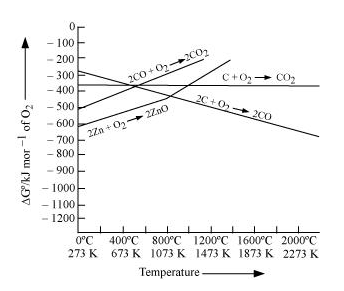ZnO is reduced to Zn at around 1673 K. From the figure below, we can observe that beyond 1073 K the Gibbs free energy of formation of CO from C and beyond 1273 K, the Gibbs free energy of formation of CO2 from C is smaller than the Gibbs free energy of formation of ZnO. Thus, C can reduce ZnO to Zn. However, the Gibbs free energy of formation of CO2 from CO is greater than the Gibbs free energy of formation of ZnO. Thus, CO is not able to reduce ZnO making C a better reducing agent for ZnO.

Question23. In some particular case thermodynamic factor dictates the choice of the reducing agent. To what extent do you believe this statement to be true ? Provide two examples to back your opinion.

Solution :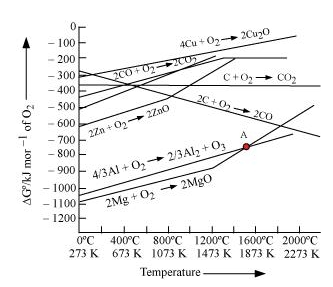The figure given below is a graph of Gibbs energy ∆Gθ vs Temperature for the formation of solid oxides. We can see from this graph that if ∆fGθ of a metal’s oxide is more negative than another metal’s oxide, then the former metal can reduce the oxide of the latter metal ( i.e., the oxide with less negative ∆fGθ ). For example, ∆fGθ ( Al, Al2O) is more negative than ∆fGθ ( Cu, Cu2O ), thus Al can readily reduce Cu2O to Cu but it is not possible for Cu to reduce Al2O3. Likewise, it isn’t possible for Zn to reduce MgO but Mg can reduce ZnO to Zn. This is because ∆fGθ ( Mg, MgO ) is more negative than ∆fGθ ( Zn,ZnO ).

Question24. What are the processes that give chlorine as a by-product ? What happens when an aqueous of NaCl is electolyzed ?

Solution :
In Down process, chlorine is obtained as a byproduct. In this process fused mixture of CaCl2 and NaCl is electrolyzed at 873 K. Sodium is obtained the cathode and Cl2 is discharged at the anode.

When an aqueous solution of NaCl is subjected to electrolysis, Cl2 is discharged at the

anode. At the cathode, His obtained ( in place of Na ). This is because the standard reduction potential of H2O (E° = − 0.83 V) is less negative than that of Na (E°= − 2.71 V). Thus, H2O will get a higher preference to get reduced at the cathode thereby discharging H2.
NaCl ⇒ Na+ + Cl
At cathode : 2H2O + 2e – ⇒ H2 + 2OH
At anode : Cl  ⇒ Cl + e
2Cl ⇒ Cl2

Question25. What role does a graphite rod play in the electrometallurgy of aluminium?

Solution :
In the electrometallurgy of aluminum, the anode is graphite and the cathode iron is graphite lined .The O2 being liberated due to electrolysis reacts with the graphite anode to liberate CO2 and CO. Had it been any other metal besides graphite, the oxygen would have reacted with the aluminum during the electrolysis to form Al2O3. This would have resulted in the wastage of aluminium and as graphite is cheaper than aluminum it is expendable.
This is the role of aluminum.

Question26. What are the principles of refining a metal by the following processes :

(a) Zone refining
(b) Electrolytic refining
(c) Vapour phase refining

Solution :
(a) Zone refining :
This method makes use of a principle according to which impurities are more soluble in molten metal than in a solid metal. In zone refining, a moving circular heater slowly moves over an impure metal rod/ bar. As the circular heater moves, the impurities move into the adjacent molten zone and the pure metal crystallizes out. This is repeated over several times, causing the segregation of impurities at one end of the bar. This impure end is then cut off. Boron, silicon, gallium etc. are purified this way.

(b) Electrolytic refining :
It is the refining of impure metals using electricity. In this method, the anode is the impure metal and the cathode is a thin sheet of pure metal. The electrolyte is a salt solution of the same metal.
On passing the electric current the metal ions from the electrolyte collect at the cathode as pure metal and the impure metal of the anode dissolves into the solution ( electrolyte ) in the form of ions. The impurities of the metal deposits below the anode. This is called the anode mud.

(c) Vapour phase refining :
It is a method of metal refining by converting the metal into its volatile compound and then decomposing it to get the pure metal.
To achieve this:
(1) the metal should convert into a volatile compound with a reagent,
(2) the volatile compound of the metal has to be able to decompose easily so that the pure metal can be conveniently recovered.
Zirconium, titanium and nickel are refined through this method.

Question27. Under what conditions could Al reduce MgO ?

Solution :
The standard Gibbs free energy of formation of Al2O3 from Al is smaller than that of MgO from Mg after 1350 0C. Thus, at a temperature beyond 1350°C, Al can reduce MgO.

Question28. Which of the ores mentioned in Table 6.1 can be concentrated by magnetic separation method?

Solution :
If the ore or the gangue can be attracted by the magnetic field, then the ore can be concentrated by the process of magnetic separation. Among the ores mentioned in table 6.1, the ores of iron such as haematite (Fe2O3), magnetite (Fe3O4), siderite (FeCO3), and iron pyrites (FeS2) can be separated by the process of magnetic separation.

Question29. What is the significance of leaching in the extraction of aluminium?

Solution :
In the extraction of aluminium, the significance of leaching is to concentrate pure alumina (Al2O3) from bauxite ore.

Bauxite usually contains silica, iron oxide, and titanium oxide as impurities. In the process of leaching, alumina is concentrated by digesting the powdered ore with a concentrated solution of NaOH at 473-523 K and 35-36 bar. Under these conditions, alumina (Al2O3) dissolves as sodium meta-aluminate and silica (SiO2) dissolves as sodium silicate leaving the impurities behind.

The impurities are then filtered and the solution is neutralized by passing CO2 gas. In this process, hydrated Al2O3 gets precipitated and sodium silicate remains in the solution. Precipitation is induced by seeding the solution with freshly prepared samples of hydrated Al2O3.

Hydrated alumina thus obtained is filtered, dried, and heated to give back pure alumina (Al2O3).

Question30. The reaction,

is thermodynamically feasible as is apparent from the Gibbs energy value. Why does it not take place at room temperature?

Solution :
The change in Gibbs energy is related to the equilibrium constant, K as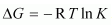.

At room temperature, all reactants and products of the given reaction are in the solid state. As a result, equilibrium does not exist between the reactants and the products. Hence, the reaction does not take place at room temperature. However, at a higher temperature, chromium melts and the reaction takes place.

We also know that according to the equation

Increasing the temperature increases the value of TΔS making the value of ΔG more and more negative. Therefore, the reaction becomes more and more feasible as the temperature is increased.

Question31. Is it true that under certain conditions, Mg can reduce SiO2 and Si can reduce MgO? What are those conditions?

Solution :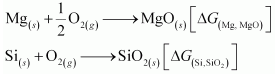The temperature range in which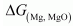is lesser than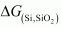, Mg can reduce SiO2 to Si.

On the other hand, the temperatures range in whichis less than, Si can reduce MgO to Mg.

The temperature at which ΔfG curves of these two substances intersect is 1966 K. Thus, at temperatures less than 1966 K, Mg can reduce SiO2 and above 1966 K, Si can reduce MgO.

Get 30% off your first purchase!

X
error: Content is protected !!
Scroll to Top